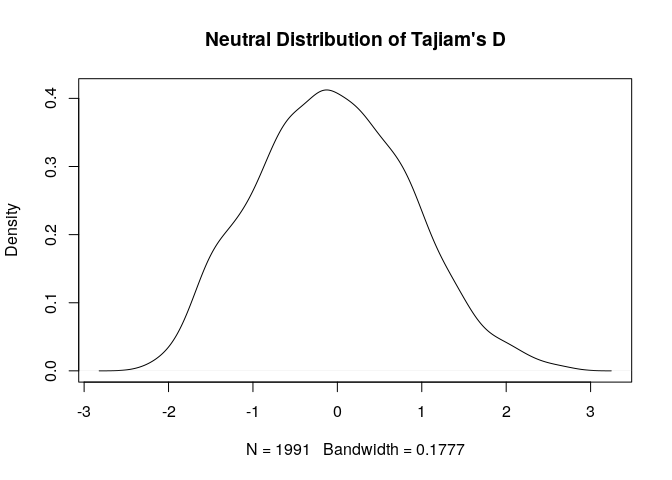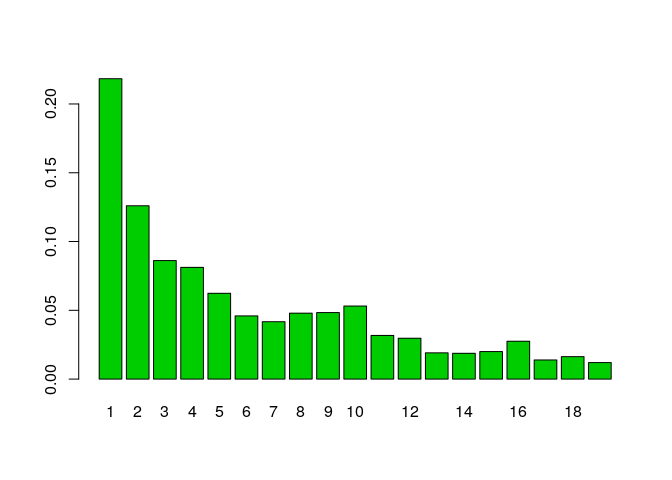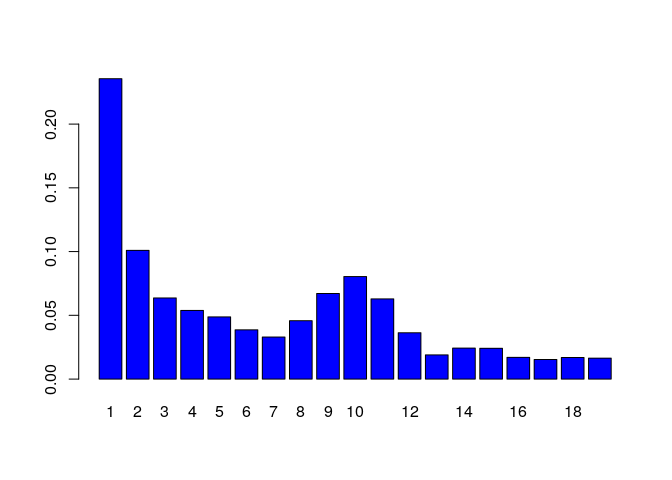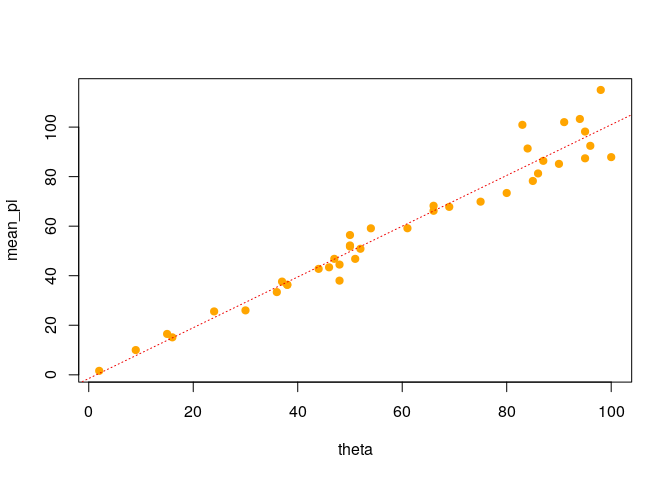Examples

1. Obtain the neutral distribution of Tajima’s D

For 20 samples from a panmictic population:

library(coala)
model <- coal_model(20, 2000) +
feat_mutation(2) +
feat_recombination(1) +
sumstat_tajimas_d()
stats <- simulate(model, seed = 15)
plot(density(stats$tajimas_d, na.rm = TRUE), main = "Neutral Distribution of Tajiam's D")2. Generate the site frequency spectrum For a neutral model with two populations and migration: model2a <- coal_model(c(10, 10), 100) + feat_mutation(10) + feat_recombination(5) + feat_migration(0.5, symmetric = TRUE) + sumstat_sfs(population = "all") stats <- simulate(model2a, seed = 20) barplot(stats$sfs / sum(stats$sfs), names.arg = seq_along(stats$sfs),
col = 3)And again, but now with a bottleneck in one population:

model2b <- model2a +
feat_size_change(0.1, population = 2, time = 0.25) +
feat_size_change(1, population = 2, time = 0.5)
stats <- simulate(model2b, seed = 25)
barplot(stats$sfs / sum(stats$sfs),
names.arg = seq_along(stats$sfs), col = 4)3. Plot the nucleotide diversity against the mutation rate: model3 <- coal_model(10, 50) + feat_mutation(par_prior("theta", sample.int(100, 1))) + sumstat_nucleotide_div() stats <- simulate(model3, nsim = 40) mean_pi <- sapply(stats, function(x) mean(x$pi))
theta <- sapply(stats, function(x) x\$pars[["theta"]])
plot(theta, mean_pi, pch = 19, col = "orange")
abline(lm(mean_pi ~ theta), col = "red2", lty = 3)If you have a nice example for using coala, feel free to extend this vignette via a pull request on GitHub!